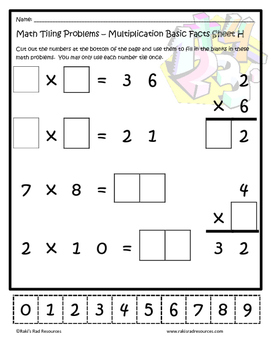# Multiplication Facts Tiling Puzzle - FREE3rd - 5th, Homeschool
Subjects
Standards
Resource Type
Formats Included
• PDF
Pages
1 page

### Description

Are you looking for a creative new way to have your students practice multiplication? Try Tiling! In Tiling activities, students will use the provided "digit tiles" to place the numbers 0 - 9 to fill in the holes left in provided multiplication equations. While trying to figure out where the missing numbers go, students will work on basic facts, properties of multiplication and problem solving skills. Simple and easy to use as a homework assignment or a math center.

Interested in using this puzzle in your Google Classroom? Check out the Google Classroom version of this product.

This is just one puzzle, which will ALWAYS remain FREE. Find a pack of 10 puzzles like this by clicking HERE .

Want more tiling puzzles? These puzzles are available in my store in PDF and Google Classroom Formats:

Basic Subtraction Facts PDF

Subtraction with Regrouping PDF

Addition & Subtraction Mixed - with Regrouping PDF

Basic Multiplication Facts PDF

Multiplying 2 digit numbers by 1 digit numbers PDF

Multiplying 2 digit numbers by 1 digit numbers Google Classroom

Multiplying 3 and 4 digit numbers by 1 digit numbers PDF

Multiplying 3 and 4 digit numbers by 1 digit numbers Google Classroom

Basic Division Facts PDF

Long Division PDF

These bundles are available in PDF format:

Addition & Subtraction Tiling Puzzle Bundle

Multiplication & Division Tiling Puzzle Bundle

Created by Heidi Raki of Raki's Rad Resources.

Total Pages
1 page
Included
Teaching Duration
1 hour
Report this Resource to TpT
Reported resources will be reviewed by our team. Report this resource to let us know if this resource violates TpT’s content guidelines.

### Standards

to see state-specific standards (only available in the US).
Fluently multiply and divide within 100, using strategies such as the relationship between multiplication and division (e.g., knowing that 8 × 5 = 40, one knows 40 ÷ 5 = 8) or properties of operations. By the end of Grade 3, know from memory all products of two one-digit numbers.
Understand division as an unknown-factor problem. For example, find 32 ÷ 8 by finding the number that makes 32 when multiplied by 8.
Apply properties of operations as strategies to multiply and divide. Examples: If 6 × 4 = 24 is known, then 4 × 6 = 24 is also known. (Commutative property of multiplication.) 3 × 5 × 2 can be found by 3 × 5 = 15, then 15 × 2 = 30, or by 5 × 2 = 10, then 3 × 10 = 30. (Associative property of multiplication.) Knowing that 8 × 5 = 40 and 8 × 2 = 16, one can find 8 × 7 as 8 × (5 + 2) = (8 × 5) + (8 × 2) = 40 + 16 = 56. (Distributive property.)
Determine the unknown whole number in a multiplication or division equation relating three whole numbers. For example, determine the unknown number that makes the equation true in each of the equations 8 × ? = 48, 5 = __ ÷ 3, 6 × 6 = ?.
Use multiplication and division within 100 to solve word problems in situations involving equal groups, arrays, and measurement quantities, e.g., by using drawings and equations with a symbol for the unknown number to represent the problem.